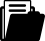Meine Position
i
Ein Tipp vom Eldar Team
Je mehr Sie bestellen, desto grösser Ihr Rabatt
Sofort lieferbarBuch
Buch
Fachbuch
2015

# Hierarchical Matrices: Algorithms and Analysis

## Algorithms and Analysis

ISBN
EAN
978-3-662-47323-8
9783662473238
Artikel-Nr.
DGZYNZ6
Sofort lieferbar:
6
Kostenloser Versand
Rabatt
-12.6
%
CHF 197.00
CHF
172.17
Anzahl
1
Maximale
Lieferzeit
5
Arbeitstage
Freitag
01.07.2022Weitere FormateBeschreibung

This self-contained monograph presents matrix algorithms and their analysis. The new technique enables not only the solution of linear systems but also the approximation of matrix functions, e.g., the matrix exponential. Other applications include the solution of matrix equations, e.g., the Lyapunov or Riccati equation. The required mathematical background can be found in the appendix.

The numerical treatment of fully populated large-scale matrices is usually rather costly. However, the technique of hierarchical matrices makes it possible to store matrices and to perform matrix operations approximately with almost linear cost and a controllable degree of approximation error. For important classes of matrices, the computational cost increases only logarithmically with the approximation error. The operations provided include the matrix inversion and LU decomposition.

Since large-scale linear algebra problems are standard in scientific computing, the subject of hierarchical matrices is of interest to scientists in computational mathematics, physics, chemistry and engineering.StichwörterZielgruppe
Research
Inhaltsverzeichnis
Preface.- Part I: Introductory and Preparatory Topics.- 1. Introduction.- 2. Rank-r Matrices.- 3. Introductory Example.- 4. Separable Expansions and Low-Rank Matrices.- 5. Matrix Partition.- Part II:  H-Matrices and Their Arithmetic.- 6. Definition and Properties of Hierarchical Matrices.- 7. Formatted Matrix Operations for Hierarchical Matrices.- 8. H2-Matrices.- 9. Miscellaneous Supplements.- Part III:  Applications.-  10. Applications to Discretised Integral Operators.- 11. Applications to Finite Element Matrices.- 12. Inversion with Partial Evaluation.- 13. Eigenvalue Problems.- 14. Matrix Functions.- 15. Matrix Equations.- 16. Tensor Spaces.- Part IV: Appendices.- A. Graphs and Trees.- B. Polynomials.- C. Linear Algebra and Functional Analysis.- D. Sinc Functions and Exponential Sums.- E. Asymptotically Smooth Functions.- References.- Index.Herausgeber/-in
Leserkritik

“Every line of the book reflects that the author is the leading expert for hierarchical matrices. … Hierarchical matrices: algorithms and analysis is without a doubt a  beautiful, comprehensive introduction to hierarchical matrices that can serve as both a graduate level textbook and a valuable resource for future research.” (Thomas Mach, Mathematical Reviews, April, 2017)

“The book ‘Hierarchical matrices: algorithms and analysis’ is a self-contained monograph which presents an efficient possibility to handle the numerical treatment of fully populated large scale matrices appearing in scientific computations, and therefore it is of interest to scientists in computational mathematics, physics, chemistry and engineering.” (Constantin Popa, zbMATH 1336.65041, 2016)

Publikation
Deutschland
21.12.2015Sprache
EnglischFormat
Buch (Hardcover)
511 SeitenVersand
Kostenlos: Schweiz & Liechtenstein
Für Deutschland oder Frankreich werden die Versandgebühren im Warenkorb berechnet.ZahlungsartenBestellen Sie einfach auf Rechnung oder bezahlen Sie bequem und gebührenfrei mit Twint, Kreditkarte, PostFinance, Apple, Google oder Samsung Pay.Passende Themen### # Mehr aus der Kollektion: Springer Series in Computational Mathematics

Kollektion abonnieren
Immer die neuste Publikation erhalten
oder
Buch einzeln bestellen
• Rabatt
-12.6
%
CHF 197.00
CHF
172.17
• Rabatt
-22.5
%
CHF 192.00
CHF
148.80
• Rabatt
-12.9
%
CHF 130.00
CHF
113.28
• Rabatt
-12.8
%
CHF 211.00
CHF
183.92
• Rabatt
-11.9
%
CHF 165.00
CHF
145.28
• Rabatt
-22.5
%
CHF 211.00
CHF
163.53Autor/-in
Zurück
Zum Start